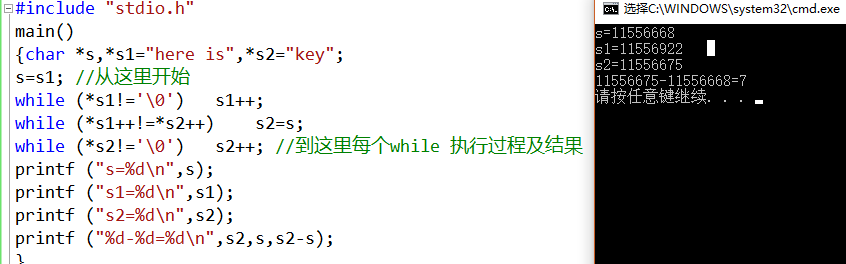• 以下程序的输出结果是： #include “stdio.h” main() {char *s,*s1=“here is”,*s2=“key”; s=s1; while (*s1!=’\0’) s1++; while (*s1++!=*s2++) s2=s; while (*s2!=’\0’) s2++; printf ("%d-%d=%d\n&...
以下程序的输出结果是：
#include “stdio.h”
main()
{char *s,*s1=“here is”,*s2=“key”;
s=s1;
while (*s1!=’\0’)	s1++;
while (*s1++!=*s2++)	s2=s;
while (*s2!=’\0’)	s2++;
printf ("%d-%d=%d\n",s2,s,s2-s);
}
之前一直不理解这个的结果是什么意思。。#include "stdio.h"
main()
{char *s,*s1="here is",*s2="key";
s=s1; //从这里开始,print的s=s1=19748668,即字符串存储起始位置
while (*s1!='\0')	s1++;//s1向后走，直到字符串的末尾\0,此时printf的s1=19748675
while (*s1++!=*s2++)	s2=s;
//这行功能：比较s1与s2，s1的“h”(位置为s+1=19748669)与s2的“k”不同，则s2=s
//这行执行后:s1=19748922,s2=19748669
while (*s2!='\0')	s2++;
//这行让s2查找至末尾\0: s2=19748675(*s2="here is")
printf ("s=%d\n",s);
printf ("s1=%d\n",s1);
printf ("s2=%d\n",s2);
printf ("%d-%d=%d\n",s2,s,s2-s);//s2-s即“here is”的长度为7
}

每次执行程序，存储位置都不一定相同，故s,s1,s2的值每次都会有所不同，但是存储的相对位置是不会改变的，s2-s即“here is”的长度，为7。


展开全文c
• 以下程序的输出结果是（ ） # include<stdio.h> subl（char a，char b） {char c；c＝a；a＝b；b=c；} sub2（char *a，char b）{ char c；c＝*a；*a＝b；b＝c；} sub3（char *a，char *b） { char c；c＝*a；*a＝*b...c
• 以下程序的输出结果是（ ）。 #include int f(int a) {  int b=0;  static int c=3;  a=c++,b++;  return a; } void main() {  int a,i,t;  a=3; ...
• 问题：从键盘上输入“446755”，以下程序的输出结果是多少 //getchar函数的应用 #include<stdio.h> int main() { int c; while((c=getchar())!='\n') //注释1 { switch(c-'2') //注释2 { case 0: //...
问题：从键盘上输入“446755”，以下程序的输出结果是多少

//getchar函数的应用
#include<stdio.h>
int main()
{
int c;
while((c=getchar())!='\n')              //注释1
{
switch(c-'2')                       //注释2
{
case 0:                         //注释3
case 1:putchar(c+4);            //注释4
case 2:putchar(c+4);break;      //注释5
case 3:putchar(c+3);            //注释6
default:putchar(c+2);break;     //注释7
}
}
printf("\n");
return 0;
}[解题思路]

1.getchar()函数：顾名思义(get character),即得到一个字符。

2.那什么是字符呢？字符常量包括普通字符和转义字符----
普通字符形如’1’、‘a’、’?’、’\$‘等用单撇号括起来的一个字符（‘1234’、‘fhssgj’这种形式表达是不正确的，对于用双撇号括起来的"1234"属于字符串常量，而不是字符常量）；
转义字符是以字符\开头的字符序列，如’\n’、’\t’、’\012’等。

3.getchar()是在输入缓冲区顺序读入一个字符(包括空格、回车和Tab)

4.getchar()会从输入缓冲区去读取内容，键盘输入字符是先存在缓冲区，当我们把所有的内容都输入完成（本例中输入446755）并且按下了Enter键后，getchar()就会进入缓冲区读取字符，每一次getchar()从输入缓冲区读取一个字符，被读取的字符从缓冲区中清除。

5.接下来我们来分析下代码：

【注释1】  当用键盘输入446755时，输入内容存在缓冲区（此getchar()还没有进入缓冲区读取字符），当按下回车键时，getchar()就会进入缓冲区读取字符，此时输入缓冲区中存在7个字符 446755\n(\n换行符是按下回车键获得的)而 getchar函数的返回值是用户输入的第一个字符的ASCII码值，字符’4’对应的ASCII码值是52，故此时整型变量c=52，进入【注释2】

【注释2】：switch后面括号里的表达式值为c-‘2’=2（字符‘2’的ASCII码值为50，52-50=2；），进入【注释5】;

【注释5】：c+4=56（注意：1.这里的4是整型，非字符；2.变量c的值仍为52，不是2，虽然注释2中有表达式c-‘2’，但未再赋给变量c），ASCII码值为56对应的字符为8；故执行putchar(c+4);后输出结果8；
putchar()函数输出的是字符，不是字符的ASCII码，如putchar(97),输出的是字符a;
这里贴上代码和结果欣赏下：

#include<stdio.h>
int main()
{
putchar(97);
return 0;
}回到本例中，输出结果8后，进入第while第2次循环，进入【注释1】，此时getchar()从缓存区读取第二个字符’4’（前面已说过，输入的第一个字符’4’已经从缓冲区清除，被用过了，还用干啥！扔了），由于第二个字符仍为’4’，故同上述步骤，经过一波操作后，屏幕上又输出8；

进入第3次循环至【注释1】， 此时getchar()从缓存区读取第3个字符’6’（字符‘6’的ASCII码值为54），满足(c=getchar())!=’\n’，代码走到【注释2】，switch后面括号里的表达式值为c-‘2’=4（字符‘2’的ASCII码值为50，54-50=4；），进入【注释7】;

【注释7】 c+2=56（注意：1.这里的2是整型，非字符；2.变量c的值仍为54，不是4，虽然注释2中有表达式c-‘2’，但未再赋给变量c），ASCII码值为56对应的字符为8；故执行putchar(c+2);后输出结果8；

再读取字符’7’……一波操作后输出9；

读取字符’5’（第一个），进入【注释6】 case 3:putchar(c+3); （注意：此语句后面没有break;,执行这条语句输出结果8，之后还没有跳出switch语句，进入注释7，接着输出7）

再读取字符’5’（第二个），同理输出结果87

最后，输入缓冲区里只剩下’\n’,不满足while（）循环条件，跳出循环，

最终输入"446755",
输出"88898787"


展开全文c语言
• public class A { public static void prt(){ System.out.println("1"); } public A(){ System.out.println("A"); } } public class B extends A{ /** ...public static void prt
public class A {public static void prt(){System.out.println("1");}public A(){System.out.println("A");}}

public class B extends A{/*** @param args*/public static void prt(){System.out.println("2");}public B(){System.out.println("B");}public static void main(String[] args) {// TODO Auto-generated method stubA a = new B();a = new A();}
}
————————————————————————
A B  A

展开全文• 输出结果是：0 要想输出结果为6，改成： import java.math.BigInteger; public class Test { public static void main(String[] args) throws NumberFormatException{ BigInteger one = ...
import java.math.BigInteger;
public class Test {public static void main(String[] args) throws NumberFormatException{BigInteger one = new BigInteger("1");BigInteger two = new BigInteger("2");BigInteger three = new BigInteger("3");BigInteger sum = new BigInteger("0");sum.add(one);sum.add(two);sum.add(three);System.out.println(sum.toString());}}

输出结果是：0
要想输出结果为6，改成：

import java.math.BigInteger;
public class Test {public static void main(String[] args) throws NumberFormatException{BigInteger one = new BigInteger("1");BigInteger two = new BigInteger("2");BigInteger three = new BigInteger("3");BigInteger sum = new BigInteger("0");sum=sum.add(one);sum=sum.add(two);sum=sum.add(three);System.out.println(sum.toString());}
}

展开全文• import java.util.*; /**  * Description:  * 网站: 疯狂Java联盟 ...------------------------------------------------------------------StringBuffer面试题-------------------------...输出结果:AB,B
• package com.njue.mis; import java.util.*; public class Test { public static void main(String[] args){ Queue queue = new PriorityQueue(10, ...public int compare(Integer i,Integer j
• 解析：联网状态下会输出4，URLequal对比方式。根据文档说明：如果两个主机名可解析为同一IP地址，则认为两个主机相同（即使主机名不等）。 "http://javapuzzlers.com", //IP地址为 208.97.154.9 ...
• 以下程序的输出结果是（ ）。 using System; namespace HoverTreeTikuConsole { class Program { static void Main(string[] args) { MyStruct s1 = new MyStruct(1, 2); s1.x = 2; s1.Sum(); ...
• #include <stdio.h> main() { int i,j; for(i=1;i<4;i++) { for(j=i;j<4;j++) printf("%d*%d=%d",i,j,i*j); printf("\n");...上边程序的输出结果是？最好详细解释下每行语句。
• void ss (char *s,char t) { while (*s) { if(*s==t) *s=t-‘a’+’A’; ...函数ss功能就是将字符串s...于主函数中函数ss调用，就是将字符串str1中小些字符’d’替换成大写字符’D’。所以 正确答案是B。
• for(i=4;i>1;i–) for(j=1;j<i;j++) putchar(’#’); 答案：###### 解析：内部循环完了之后再进行外部循环，第二次外部循环时候...putchar:c语言函数之一，作用是向终端输出一个字符,这里一共循环输出6次 ...
• main() { int p={11,13,14,15,16,17,18}; int i=0,j=0; while(i<7 && p[i]%2==1) j+=p[i++];...printf("%d\n",j);...i等于0时,p为奇数，进入while循环，j累加了p值为11; i加1为1...
• 输出的结果是3个3，因为在主程序中遇到异步事件时候，会将异步事件放到事件队列里面去，等到主程序执行完之后，在去执行事件队列里面事件，此时主程序已经执行完，i变为3，由于每次输出都是i值，所以是3个3 ...javascript
• #include #include using namespace std; int main(void) { int i,j,k,l; cout<<“Input i:”<<endl; cin>>oct>>i; //输入为八进制数 cout<<“hex:”<<...}c++
• 以下程序的输出结果是_ main) { float a ; a=1/100000000 ; printf%g,a; } 下面程序的输出结果是_ #include<stdio.h> main) { int x=10 ; { int x=20 ; printf%d,x; } printf%d\n,x; } 以下程序的输出结果是_ main) ...
• #include <stdio.h> int main (int argc, char *argv[]) { int a = {(1,2),(3,4),(5,6)}; int *p = a; printf("%d\n",p);...输出答案为：2 int a = {(1,2),(3,4),(5,6)}; 上面赋值...
•java
• 例子1：   静态方法优先于主方法，且执行一次 构造块，只要建立一个对象就会执行一次 输出结果： static block instance1 block instance2     例子2： ...
• 以下程序的功能是输入一个字符串和一个字符，如果该字符在字符串中，就从该字符首次出现的位置开始输出字符串中的字符。例如，如果输入的字符串为“Hello”，输入的字符为’e’，则输出结果为“ello”。请填空。 ...c语言 指针
• import java.util.Scanner; public class Work0225_8 { public static void main(String[] args) { ...以下图片是控制台输出的结果： ![图片说明](https://img-ask.csdn.net/upload/201602/25/1456401752_905094.png)
• 已知字符AASCⅡ代码值为65，以下程序运行时若从键盘输入：B33<回车>，则输出结果是？ #include<stdio.h> main() { char a,b; a=getchar(); scanf("%d",&b); a=a-'A'+'O'; b=b*2; printf...
• 给定以下方法声明，调用执行 mystery（1234） 的输出结果？ (43211234) //precondition: x >=0 public void mystery (int x) { System.out.print(x % 10); if ((x / 10) != 0){ mystery(x / 10); } ...java
• 以下是该无聊的程序: #include <stdio.h> #include <string.h> char* get_str() { int x; char str[] = "hello world! long long long long long long long!!!"; retur......